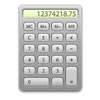﻿ Calculators# Calculators

Here are some (hopfully useful) calculators which I've written. Having never done anything with Javascript before, here's a brief example. Note that Javascript commands are case sensitive.

In the <head> tag of the page, put the following:

```<script language="javascript">
function calc_capacitance(dist,area,perm)
{
return (1e9*perm*8.854e-12*(area/10000)/(dist/1000)).toFixed(3);
}
</script>
```

This provides a function calc_capacitance() which returns the capacitance of parallel plates (in nF), given the distance between them in mm, the area in cm^2, and the relative permittivity. The function is self-explanatory; the only odd thing is .toFixed(3). This truncates the result to a specified number of decimal places (see http://www.w3schools.com/jsref/jsref_tofixed.asp).

Next, create four text input fields on the page, like this (obviously, separate them out to make them easier to identify):

```<input name="txt_dist" type="text" id="txt_dist" />
<input name="txt_area" type="text" id="txt_area" />
<input name="txt_perm" type="text" id="txt_perm" />
<input name="txt_cap" type="text" id="txt_cap" />
```

There is a subtle distinction between the name and id attirbutes (name is unique to a form, id must be unique on the page), but make them both the same. Call the text fields txt_dist, txt_area, txt_perm, txt_cap.

To get the numerical value of something entered in a text field, we use

```parseFloat(document.getElementById('txt_dist').value)
```

Note the single quotes around txt_dist. document.getElementById('txt_dist').value returns a string value, and parseFloat() turns that into a floating-point numerical value.

To display something in a text field, we can use one of the following

```document.getElementById('txt_cap').value='hello'
document.getElementById('txt_cap').value=123.45
document.getElementById('txt_cap').value=numerical_result```

(depending on whether it's a string constant, numerical constant, or numerical variable we want to display.) If we wanted to display the result of our calc_capacitance() function, we could do

`document.getElementById('txt_cap').value=calc_capacitance(1,20,2)`

which would return a value of 0.035nF. How do we create a button to do this when clicked? Simple (note that I have split this into several lines for readability. It must all be on one line, so remove the underscores):

```<input name="btn_cap" type="button" id="btn_cap" value="Calculate capacitance" _
onclick="document.getElementById('txt_cap').value= _
calc_capacitance(parseFloat(document.getElementById('txt_dist').value), _
parseFloat(document.getElementById('txt_area').value), _
parseFloat(document.getElementById('txt_perm').value));"/>```

This creates a button (remember to set the name and id to something unique on the page). When clicked, the code contained in onclick is executed, so we just stick our Javascript code into there and this fills the txt_cap text field with the result of the calculation. Have a look at the source code of the calculator pages and you'll get a feel for what's happening.

Right, the calculators (opens in new tab, and without any fancy formatting):

## Energy stored in capacitor

(Note to self: Use 150dpi for equation images)